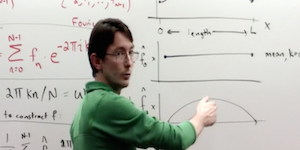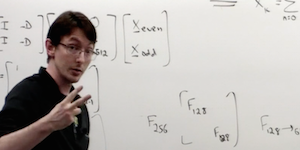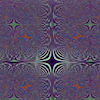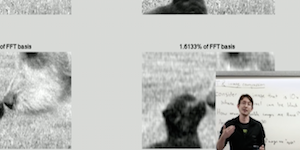# Beginning Scientific Computing

## Fourier Analysis

### Lecture 1### [ view ]

THEORY OF THE FOURIER TRANSFORM: Outline of the basic theory of the Fourier Transform and the representation of data in the frequency domain.

MATLAB COMMANDS

`FFT`

### Lecture 2### [ view ]

DISCRETE FOURIER TRANSFORM (DFT) AND THE FAST FOURIER TRANSFORM (FFT): This lecture details the algorithm used for constructing the FFT and DFT representations using efficient computation.

MATLAB COMMANDS

`FFT DFT DCT DST`

MATLAB CODE

### Supplementary VideosView
Discrete Fourier Transform

### Lecture 3### [ view ]

FFT AND IMAGE COMPRESSION: The applications of the FFT are immense. Here it is shown to be useful in compressing images in the frequency domain.

MATLAB COMMANDS

`FFT2`

MATLAB CODE

© 2015 kutz